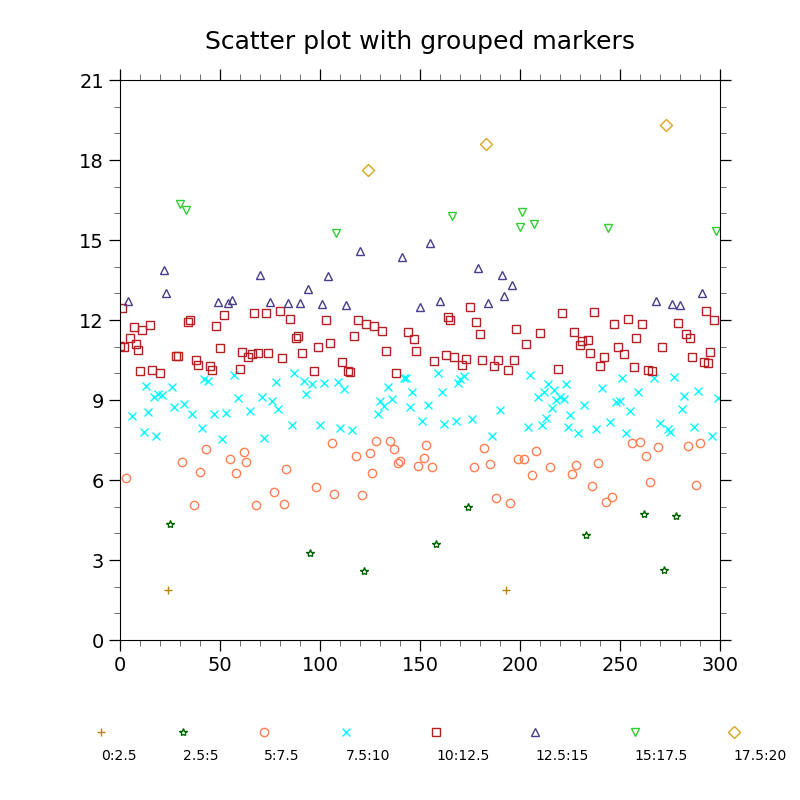# NCL_scatter_5.py#

This script illustrates the following concepts:
• Drawing a scatter plot with markers of different colors

• Generating dummy data using “random.normal”

• Manually creating a legend using markers and text

• Customizing the label locations in a legend

• Changing the orientation of a legend

• Drawing a legend outside an XY plot

• Changing the markers in an XY plot

• Changing the marker color in an XY plot

• Changing the marker size in an XY plot

See following URLs to see the reproduced NCL plot & script:

Import packages:

```import numpy as np
import matplotlib.pyplot as plt
from cycler import cycler

import geocat.viz as gv
```

Generate random data from a normal (Gaussian) distribution with a mean of 10 and standard deviation of 3

```npts = 300
random = np.random.default_rng(seed=1)
data = random.normal(loc=10, scale=3, size=npts)
```

Specify colors and markers

```colors = [
'darkgoldenrod', 'darkgreen', 'coral', 'cyan', 'firebrick', 'darkslateblue',
'limegreen', 'goldenrod'
]
markers = ['+', '*', 'o', 'x', 's', '^', 'v', 'D']

# This line cycles which color is used to plot the markers
plt.rcParams['axes.prop_cycle'] = cycler(color=colors)
```

Plot

```fig = plt.figure(figsize=(8, 8))
# Adjust the axes size to accommodate the legend at the bottom
ax = plt.axes([0.15, 0.2, 0.75, 0.70])

# Divide data into 8 bins and plot
numBins = 8
indices = np.arange(0, 300)
partitions = np.linspace(0, 20, numBins + 1)
label = "{start:g}:{end:g}"
for x in range(0, numBins):
bins = np.where(data > partitions[x], data, np.nan)
with np.errstate(
invalid='ignore'
):  # Indeed not needed, just to get rid of warnings about numpy's NaN comparisons
bins = np.where(bins < partitions[x + 1], bins, np.nan)
indices = np.where(bins != np.nan, indices, np.nan)
plt.plot(indices,
bins,
marker=markers[x],
fillstyle='none',
linewidth=0,
label=label.format(start=partitions[x], end=partitions[x + 1]))

# `ncol` being equal to the number of labels makes it appear horizontal
legend = ax.legend(bbox_to_anchor=(-0.075, -0.2),
ncol=numBins,
loc='lower left',
columnspacing=0.5,
frameon=False)
for txt in legend.get_texts():
txt.set_ha("center")  # horizontal alignment of text item
txt.set_va("center")  # vertical alignment of text item
# Move label text so it is centered under the marker
txt.set_x(-25)  # x-position
txt.set_y(-20)  # y-position

# Use geocat.viz.util convenience function to set axes parameters
gv.set_axes_limits_and_ticks(ax,
xlim=(0, 300),
ylim=(0, 21),
xticks=range(0, 301, 50),
yticks=range(0, 22, 3))

# Use geocat.viz.util convenience function to add minor and major tick lines
x_minor_per_major=5,
y_minor_per_major=3,
labelsize=14)

# Use geocat.viz.util convenience function to set titles and labels
gv.set_titles_and_labels(ax, maintitle="Scatter plot with grouped markers")

plt.show()
```Total running time of the script: ( 0 minutes 0.183 seconds)

Gallery generated by Sphinx-Gallery